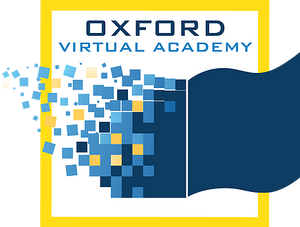# Precalculus

This course presents students with a formal study of functions, an analysis of sequences and series, counting principles, the binomial theorem, and probability. Students will use technology to employ multiple approaches to problem solving and data modeling. This course also includes topics on trigonometry, parametric curves, the polar coordinate system, and complex numbers in polar form. Students will solve problems using the Laws of Sines and Cosines and will also analyze vectors and conics, study systems of equations and matrices, and solve systems using matrices. Limits and continuity are introduced.

* Prerequisites: Algebra II